# IBPS PO Quantitative Aptitude Quiz- 16

## IBPS PO Quantitative Aptitude Quiz

Quantitative Aptitude is a very essential and scoring section of almost every competitive exam. To help you all score better in the exams, we are providing you with the IBPS PO Quantitative Aptitude Quiz. In this IBPS PO Quantitative Aptitude Quiz, candidates will be given a full explanation of each question. This IBPS PO Quantitative Aptitude Quiz contains a variety of questions ranging from easy to difficult level. This IBPS PO Quantitative Aptitude Quiz includes all of the most recent pattern-based questions, as well as Previous Year Quantitative Aptitude Questions from banking exams. This IBPS PO Quantitative Aptitude Quiz is available to you at no cost. This IBPS PO Quantitative Aptitude Quiz will assist aspirants in better understanding the topic and learning how to solve tricky problems. Candidates must try to solve this IBPS PO Quantitative Aptitude Quiz to enhance their preparation for upcoming exams.

Directions (1-5): Study the given table carefully to answer the following questions.
In the given table there are five colleges in which total student and percentage of engineering students and ratio of arts and commerce students are given.
There are only three types of streams in each college.
Note → some data are missing, calculate the missing data if necessary.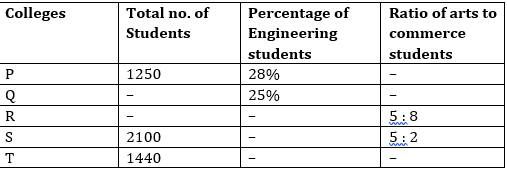1. If the ratio of boys and girls in college P for commerce student is 2 : 5 and the commerce student are 40% more than arts student. Then find the difference of boys and girls in Commerce?
(a) 225
(b) 275
(c) 250
(d) 325
(e) None of these2. If the total engineering student in college T is 360 and student in arts are 25% more than the student in commerce and engineering student in college S is 630. Then find ratio of arts student in college T and S?
(a) 2 : 3
(b) 4 : 7
(c) 4 : 9
(d) 7 : 4
(e) None of these3. If Engineering students in college P is 150 less than engineering student in college Q. Then total student in college S is what percent more or less than total student in college Q?
(a) 1%
(b) 3%
(c) 9%
(d) 7%
(e) 5%4. If total student in college R is 2600 and total engineering student in college R is equal to the total students in arts and commerce. And ratio of boys and girls in college R in engineering 5 : 8. If 20% of boys are transferred to college T. Then find total student in college T?
(a) 1640
(b) 1840
(c) 1920
(d) 1540
(e) None of these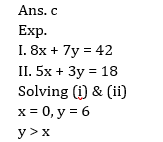5. Suppose there is another college A in which engineering students are 2/5th of the engineering student of college P and arts student in college A is 100/3% more than engineering student in college Q . And commerce student in college A is 25% more than commerce student of college S. [Given that engineering student in college S is 700 and total student is called Q is 1260].
(a) 1120
(b) 1020
(c) 1060
(d) 1080
(e) None of these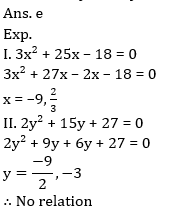Directions (6-10): In the following questions two equations numbered (I) and (II) are given. You have to solve both equations and Give answer
6. I. x² – 25x + 156 = 0
II. y² – 21y + 108 = 0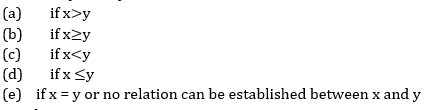7. I. 2x² – 7x – 15 = 0
II. 2y² +15y + 25 = 0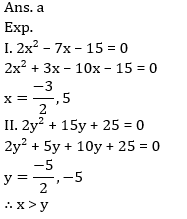8. I. 20x² + 27x + 9 = 0
II. 6y² + 29y + 35 = 09. I. 8x + 7y = 42
II. 5x+ 3y = 1810. I. 3x² + 25x – 18 = 0
II. 2y² + 15y + 27 = 0###Click to Buy Bank MahaCombo Package

Recommended PDF’s for:

#### Most important PDF’s for Bank, SSC, Railway and Other Government Exam : Download PDF Now

AATMA-NIRBHAR Series- Static GK/Awareness Practice Ebook PDF Get PDF here
The Banking Awareness 500 MCQs E-book| Bilingual (Hindi + English) Get PDF here
AATMA-NIRBHAR Series- Banking Awareness Practice Ebook PDF Get PDF here
Computer Awareness Capsule 2.O Get PDF here
AATMA-NIRBHAR Series Quantitative Aptitude Topic-Wise PDF Get PDF here
AATMA-NIRBHAR Series Reasoning Topic-Wise PDF Get PDF Here
Memory Based Puzzle E-book | 2016-19 Exams Covered Get PDF here
Caselet Data Interpretation 200 Questions Get PDF here
Puzzle & Seating Arrangement E-Book for BANK PO MAINS (Vol-1) Get PDF here
3